# How to use Excel IRR function to calculate internal rate of return

This tutorial explains the syntax of the Excel IRR function and shows how to use an IRR formula to calculate the internal rate of return for a series of annual or monthly cash flows.

IRR in Excel is one of the financial functions for calculating the internal rate of return, which is frequently used in capital budgeting to judge projected returns on investments.

## IRR function in Excel

The Excel IRR function returns the internal rate of return for a series of periodic cash flows represented by positive and negative numbers.

In all calculations, it's implicitly assumed that:

• There are equal time intervals between all cash flows.
• All cash flows occur at the end of a period.
• Profits generated by the project are reinvested at the internal rate of return.

The function is available in all versions of Excel for Office 365, Excel 2019, Excel 2016, Excel 2013, Excel 2010 and Excel 2007.

The syntax of the Excel IRR function is as follows:

IRR(values, [guess])

Where:

• Values (required) – an array or a reference to a range of cells representing the series of cash flows for which you want to find the internal rate of return.
• Guess (optional) – your guess at what the internal rate of return might be. It should be provided as a percentage or corresponding decimal number. If omitted, the default value of 0.1 (10%) is used.

For example, to calculate IRR for cash flows in B2:B5, you'd use this formula:

`=IRR(B2:B5)`

For the result to display correctly, please make sure the Percentage format is set for the formula cell (usually Excel does this automatically).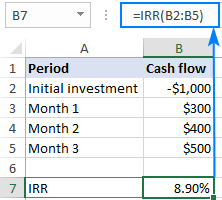As shown in the above screenshot, our Excel IRR formula returns 8.9%. Is this rate good or bad? Well, it depends on several factors.

Generally, a calculated internal rate of return is compared to a company's weighted average cost of capital or hurdle rate. If the IRR is higher than the hurdle rate, the project is considered a good investment; if lower, the project should be rejected.

In our example, if it costs you 7% to borrow money, then an IRR of about 9% is fairly good. But if the cost of funds is, say 12%, then the IRR of 9% is not good enough.

In reality, there are many other factors that influence an investment decision such as the net present value, absolute return value, etc. For more information, please see IRR basics.

### 5 things you should know about Excel IRR function

To ensure that your IRR calculation in Excel is done correctly, please remember these simple facts:

1. The values argument must contain at least one positive value (representing income) and one negative value (representing outlay).
2. Only numbers in the values argument are processed; text, logical values, or empty cells are ignored.
3. The cash flows do not necessarily have to be even, but they must occur at regular intervals, for example monthly, quarterly or annually.
4. Because IRR in Excel interprets the order of cash flows based on the order of values, the values should be in chronological order.
5. In most situations, the guess argument is not really needed. However, if the IRR equation has more than one solution, the rate closest to the guess is returned. So, your formula produces an unexpected result or a #NUM! error, try a different guess.

## Understanding IRR formula in Excel

Since the internal rate of return (IRR) is a discount rate that makes the net present value (NPV) of a given series of cash flows equal to zero, the IRR calculation relies on the traditional NPV formula: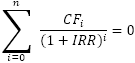Where:

• CF - cash flow
• i - period number
• n - periods total
• IRR - internal rate of return

Because of a specific nature of this formula, there is no way to calculate IRR other than through trial and error. Microsoft Excel also relies on this technique but does multiple iterations extremely quickly. Starting with the guess (if supplied) or the default 10%, the Excel IRR function cycles through the calculation until it finds the result accurate within 0.00001%. If after 20 iterations an accurate result is not found, the #NUM! error is returned.

To see how it works in practice, let's perform this IRR calculation on a sample data set. For starters, we will try to guess what the internal rate of return might be (say 7%), and then work out the net present value.

Assuming B3 is the cash flow and A3 is the period number, the following formula gives us the present value (PV) of the future cash flow:

`=B3/(1+7%)^A3`

Then we copy the above formula to other cells and add up all the present values, including the initial investment:

`=SUM(C2:C5)`

And find out that at 7% we get the NPV of \$37.90: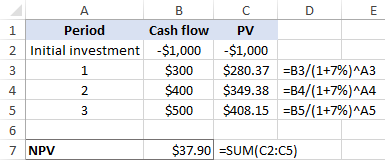Obviously, our guess is wrong. Now, let's do the same calculation based on the rate computed by the IRR function (around 8.9%). Yep, it does lead to a zero NPV: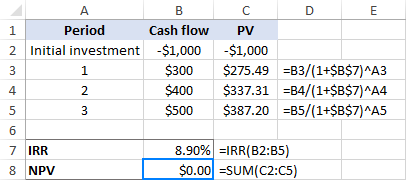Tip. To display the exact NPV value, choose to show more decimal places or apply the Scientific format. In this example, the NPV is exactly zero, which is a very rare case!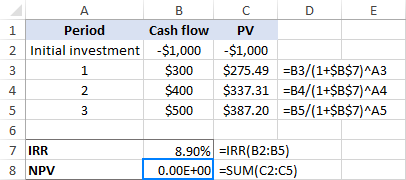## Using IRR function in Excel – formula examples

Now that you know the theoretical basis of IRR calculation in Excel, let's make a couple of formulas to see how it works in practice.

### Example 1. Calculate IRR for monthly cash flows

Assuming you have been running business for six months and now you want to figure out the rate of return for your cash flow.

Finding IRR in Excel is very straightforward:

1. Type the initial investment into some cell (B2 in our case). Since it's an outgoing payment, it needs to be a negative number.
2. Type the subsequent cash flows into the cells under or to the right of the initial investment (B2:B8 in this example). This money has been coming in through sales, so we enter these as positive numbers.

Now, you are ready to calculate IRR for the project:

`=IRR(B2:B8)`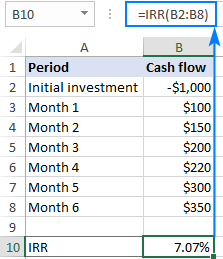Note. In event of monthly cash flows, the IRR function produces a monthly rate of return. To get an annual rate of return for monthly cash flow, you can use the XIRR function.

### Example 2: Use guess in Excel IRR formula

Optionally, you can put an expected internal rate of return, say 10 percent, in the guess argument:

`=IRR(B2:B8, 10%)`

As shown in the screenshot below, our guess does not have any impact on the result. But in some cases, changing the guess value may cause an IRR formula to return a different rate. For more information, please see Multiple IRRs.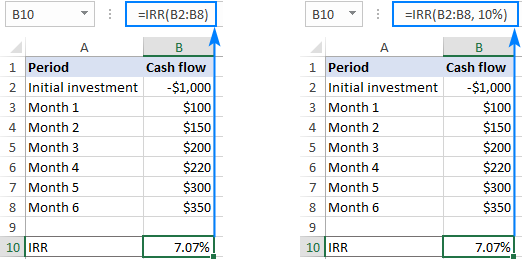### Example 3. Find IRR to compare investments

In capital budgeting, IRR values are often used to compare investments and rank projects in terms of their potential profitability. This example demonstrates the technique in its simplest form.

Supposing you have three investment options and you are deciding which one to choose. The reasonably projected returns on the investments can help you make an informed decision. For this, enter the cash flow for each project in a separate column, and then calculate the internal rate of return for each project individually:

Formula for project 1:

`=IRR(B2:B7)`

Formula for project 2:

`=IRR(C2:C7)`

Formula for project 3:

`=IRR(D2:D7)`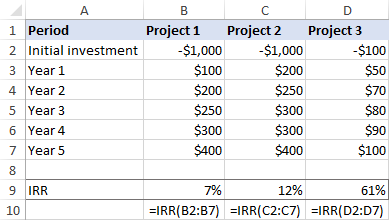Given that the company's required rate of return is, say 9%, project 1 should be rejected because its IRR is only 7%.

The two other investments are acceptable because both can generate an IRR higher than the company's hurdle rate. Which one would you choose?

At first sight, project 3 looks more preferable because it has the highest internal rate of return. However, its yearly cash flows are much lower than for project 2. In situation when a small investment has a very high rate of return, businesses often choose an investment with a lower percentage return but higher absolute (dollar) return value, which is project 2.

The conclusion is: the investment with the highest internal rate of return is usually preferred, but to make the best use of your funds you should evaluate other indicators as well.

### Example 4. Calculate compound annual growth rate (CAGR)

Though the IRR function in Excel is designed for calculating the internal return rate, it can also be used for computing the compound growth rate. You will just have to reorganize your original data in this way:

• Keep the first value of the initial investment as a negative number and the ending value as a positive number.
• Replace the interim cash flow values with zeros.

When done, write a regular IRR formula and it will return the CAGR:

`=IRR(B2:B8)`

To make sure the result is correct, you can verify it with the commonly used formula for calculating CAGR:

(end_value/start_value)^(1/no. of periods) -1

As shown in the screenshot below, both formulas produce the same result: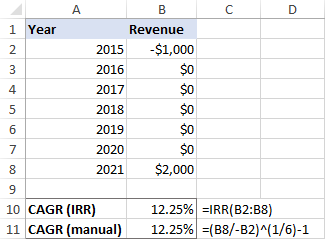## IRR and NPV in Excel

The internal rate of return and the net present value are two closely related concepts, and it's impossible to fully understand IRR without understanding NPV. The result of IRR is nothing else but the discount rate corresponding to a zero net present value.

The essential difference is that NPV is an absolute measure that reflects the dollar amount of value that could be gained or lost by undertaking a project, while IRR is the percentage rate of return expected from an investment.

Because of their different nature, IRR and NPV may "conflict" with each other - one project may have a higher NPV and the other a higher IRR. Whenever such a conflict arises, finance experts advise to favor the project with a higher net present value.

To better understand the relationship between IRR and NPV, please consider the following example. Let's say, you have a project that requires an initial investment of \$1,000 (cell B2) and a discount rate of 10% (cell E1). The project's lifespan is five years and the expected cash inflows for each year are listed in cells B3:B7.

To find out how much the future cash flows are worth now, we need to compute the net present value of the project. For this, use the NPV function and subtract the initial investment from it (because the initial investment is a negative number, the addition operation is used):

`=NPV(E1,B3:B7)+B2`

A positive net present value indicates that our project is going to be profitable: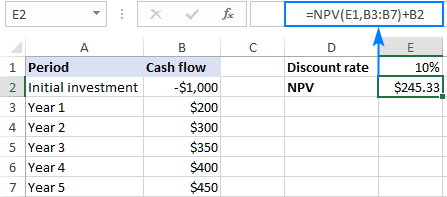What discount rate will make the NPV equal to zero? The following IRR formula gives the answer:

`=IRR(B2:B7)`

To check this, take the above NPV formula and replace the discount rate (E1) with IRR (E4):

`=NPV(E4,B3:B7)+B2`

Or you can embed the IRR function directly into the rate argument of NPV:

`=NPV(IRR(B2:B7),B3:B7)+B2`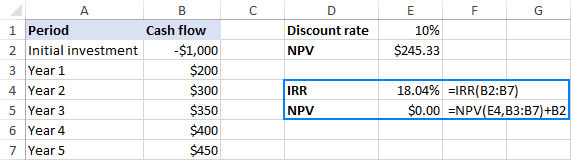The screenshot above shows that the NPV value rounded to 2 decimal places indeed equals zero. If you are curious to know the exact number, set the Scientific format to the NPV cell or choose to show more decimal places: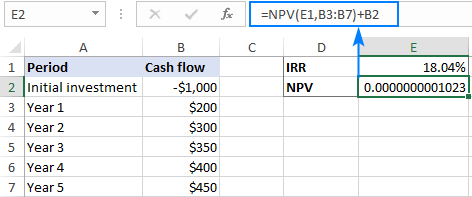As you can see, the result is well within the declared accuracy of 0.00001 percent, and we can say that the NPV is effectively 0.

Tip. If you do not entirely trust the result of IRR calculation in Excel, you can always check it by using the NPV function like shown above.

## Excel IRR function not working

If you have run into some problem with IRR in Excel, the following tips might give you a clue for fixing it.

### IRR formula returns a #NUM! error

A #NUM! error may be returned because of these reasons:

• The IRR function fails to find the result with up to 0.000001% accuracy on the 100th try.
• The supplied values range does not contain at least one negative and at least one positive cash flow.

### Blank cells in the values array

In case no cash flow arises in one or more periods, you may end up with empty cells in the values range. And it is the source of problems because rows with empty cells are left out of Excel IRR calculation. To fix this, just enter zero values in all blank cells. Excel will now see the correct time intervals and compute the internal return rate correctly.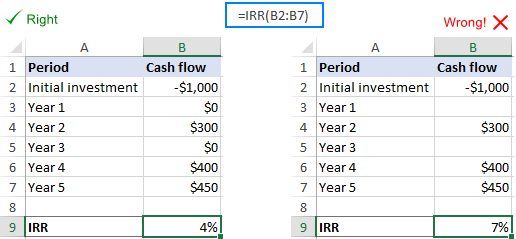### Multiple IRRs

In situation when a cashflow series changes from negative to positive or vice versa more than once, multiple IRRs can be found.

If the result of your formula is far from what you expected, check the guess value – in case the IRR equation can be solved with several rate values, the rate closest to the guess is returned.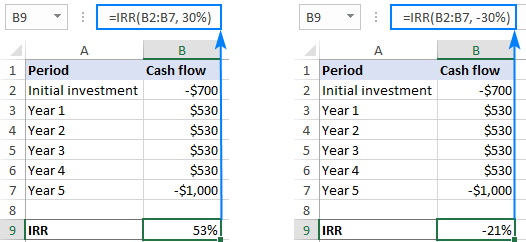Possible solutions:

• Assuming that you know what kind of return you are expecting from a certain investment, use your expectation as a guess.
• When you get more than one IRR for the same cash flow, choose the one closest to your company's cost of capital as the "true" IRR.
• Use the MIRR function to avoid the problem of multiple IRRs.

### Irregular cash flow intervals

The IRR function in Excel is designed to work with regular cash flow periods such as weekly, monthly, quarterly or annually. If your inflows and outflows occur at unequal intervals, IRR would still consider the intervals equal and return a wrong result. In this case, use the XIRR function instead of IRR.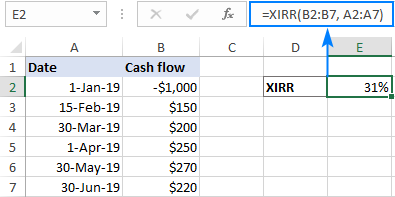### Different borrowing and reinvestment rates

The IRR function implies that the project earnings (positive cash flows) are continuously reinvested at the internal rate of return. But in the real word, the rate at which you borrow money and the rate at which you reinvest the profits are often different. Luckily for us, Microsoft Excel has a special function to take care of this scenario – the MIRR function.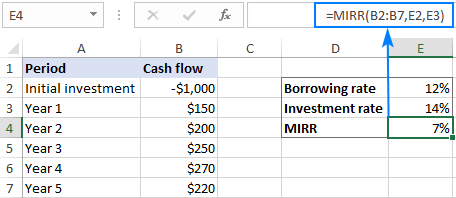That's how to do IRR in Excel. To have a closer look at the examples discussed in this tutorial, you are welcome to download our sample workbook below. I thank you for reading and hope to see you on our blog next week!

Using IRR function in Excel - examples (.xlsx file)

## You may also be interested in

1. Dear Svetlana,

I have a question related to finance. I have a row with net profits forecasted for 60 months in row 62 (B62:BI62). I want to calculate the need of funds. Usually the way to do it is to use the formula (SUMIF(B62:BI62,"<0")) . However, profits switch back and forth between positive and negative numbers and at a certain point the positive profits outweigh the negative profits. Thus using the (SUMIF(B62:BI62,"<0")) function would overestimate the need of funds. Is there a way around this problem? Thank you

2. Hello Svetlana,

Overall your article is excellent and clear, thank you.

A quick clarification and question about the IRR formula you provide in the "Understanding IRR formula in Excel" section, namely, I think the formula should be stated as:

NPV = Sum from i=0 to n of CF_i / (1 + IRR)^i = 0

In other words, NPV should be on the far left-hand side, not IRR, since we are iteratively solving for IRR for which the NPV equals zero per the definition you state in the opening paragraph:

"...the internal rate of return (IRR) is a discount rate that makes the net present value (NPV) of a given series of cash flows equal to zero"

Do you concur? If not, what are your reasons?

If you do concur, it seems like the IRR formulas in the companion page, "How to calculate IRR in Excel with formulas, template and Goal Seek" (https://www.ablebits.com/office-addins-blog/calculate-irr-excel/#what-is-irr), should also reflect this change.

Also, in your subsequent Excel formula:

=B3/(1+\$B\$7)^A3

I believe "\$B\$7" (without quotes) should actually be 7% as you state in the ensuing Excel screenshot, since 7% is the assumed IRR though cell \$B\$7 maps to the sum of the PVs which would then make the above stated formula circular.

Thank you in advance for clarifying. Regards, Fenix

• Hello Fenix,

Thank you very much for your thoughtful feedback! I agree with both points.

\$B\$7 is definitely a mistake. Sorry for that, fixed.

As for the generic IRR formula, perhaps it would be better to remove the leftmost part altogether, not to confuse inexperienced users.

Again, thank you for posting your observations!

3. It is easily described. Many thanks.

Best Regards
Abdus Sattar

4. Hi, i was wondering how do i calculate IRR mid way in an investment. thanks

5. Thanks for the tutorial
Michael Kintu , Uganda

6. how do you calculate irr if the return is the same each year for ever

7. Svetlana, an interesting additional point to include in your things to know section... Excel takes the first flow as investment/expenditure, and the rest of them with the same sign as well, whether positive or negative, opposing flows in sign are then treated as income... kind of logically, since hard to explain a project producing money before investing any... but at the same time, erroneously. Therefore, the following two flows (-100,120) and (100,-120) yield the same 20% IRR which would certainly be incorrect, but it seems that is the way Excel autocorrects. So careful with that first flow. Thought might complete your article nicely.

• Thank you for this information, Rodrigo. That's a very interesting point!

8. Hi, I have a question regarding IRR on Excel! I'm calculating the free cash flows across six periods (year 0-year 5), and all of my free cash flows end up being negative. Does this mean Excel won't calculate the IRR? Can I even have an IRR?